# Interior Angles

Interior Angles
Go back to  'Angles'

 1 What are Interior Angles? 2 Sum of Interior Angles Formula (with illustration) 3 Finding an Unknown Interior Angle 4 Finding the Interior Angles of Regular Polygons (with illustration) 5 Alternate Interior Angle Theorem (with illustration) 6 Co-Interior Angle Theorem (with illustration) 7 Important Notes on Interior Angles 8 Solved Examples on Interior Angles 9 Challenging Questions on Interior Angles 10 Practice Questions on Interior Angles 11 Maths Olympiad Sample Papers 12 Frequently Asked Questions (FAQs) 13 Download FREE Worksheets of Interior Angles

We at Cuemath believe that Math is a life skill. Our Math Experts focus on the “Why” behind the “What.” Students can explore from a huge range of interactive worksheets, visuals, simulations, practice tests, and more to understand a concept in depth.

Book a FREE trial class today! and experience Cuemath's LIVE Online Class with your child.

## What are Interior Angles?

We can define interior angles in two ways.

1. The angles that lie inside a shape (generally a polygon) are said to be interior angles.

Example: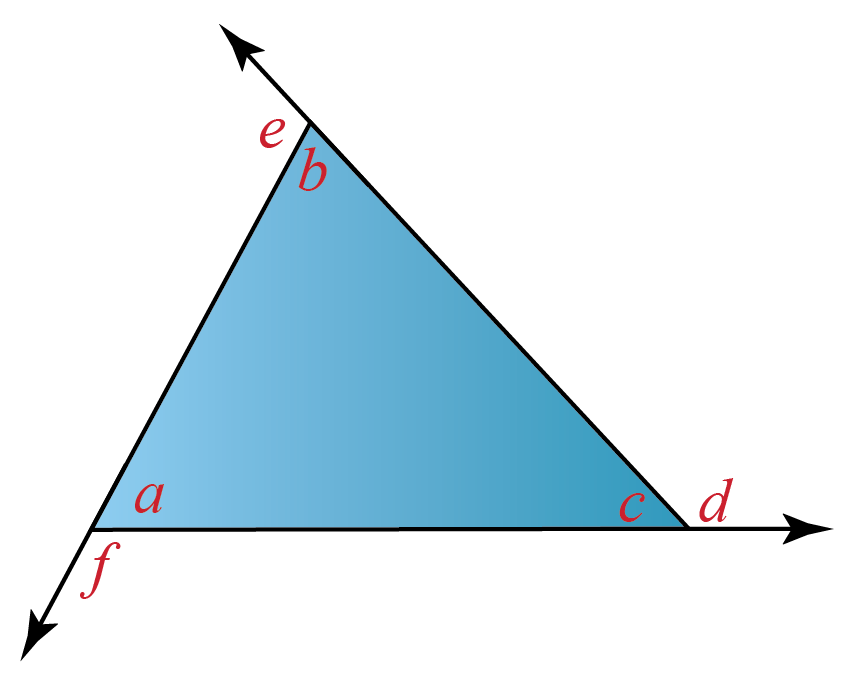In the above figure, the angles $$a, b$$ and $$c$$ are interior angles.

The angles $$d, e$$ and $$f$$ are called exterior angles.

2. The angles that lie in the area enclosed between two parallel lines that are intersected by a transversal are also called interior angles.

Example: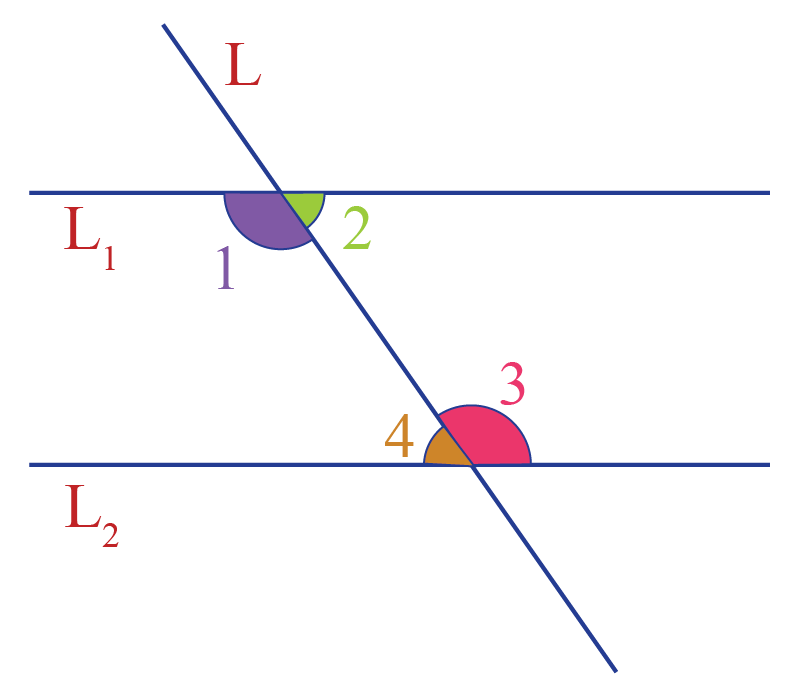In the above figure, $$L_1$$ and $$L_2$$ are parallel and $$L$$ is the transversal.

Here, the angles 1, 2, 3 and 4 are interior angles.

### Alternate Interior Angles

Alternate interior angles are the pair of non-adjacent interior angles that lie on the opposite sides of the transversal.

In the above figure, the pairs of alternate interior angles are:

• 1 and 3

• 2 and 4

### Co-Interior Angles

Co-interior angles are the pair of non-adjacent interior angles that lie on the same side of the transversal.

In the above figure, the pairs of co-interior angles are:

• 1 and 4

• 2 and 3

## Sum of Interior Angles Formula (with Illustration)

We know that the sum of all the three interior angles of a triangle is 180$$^\circ$$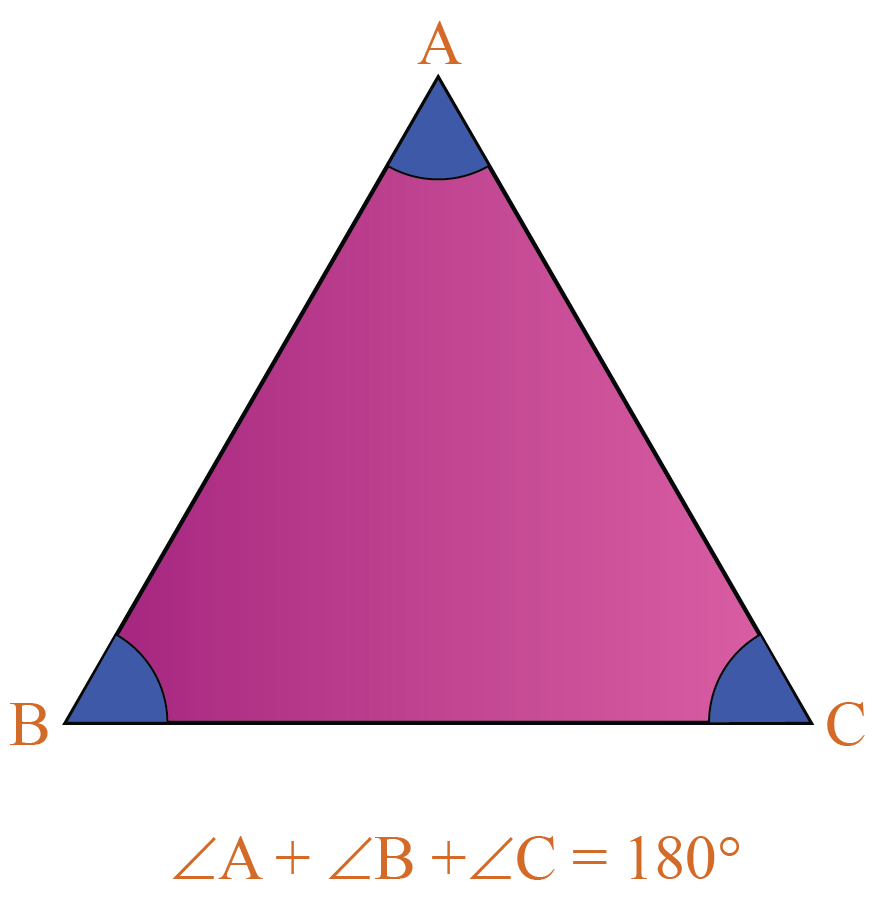We also know that the sum of all the four interior angles of any quadrilateral is 360$$^\circ$$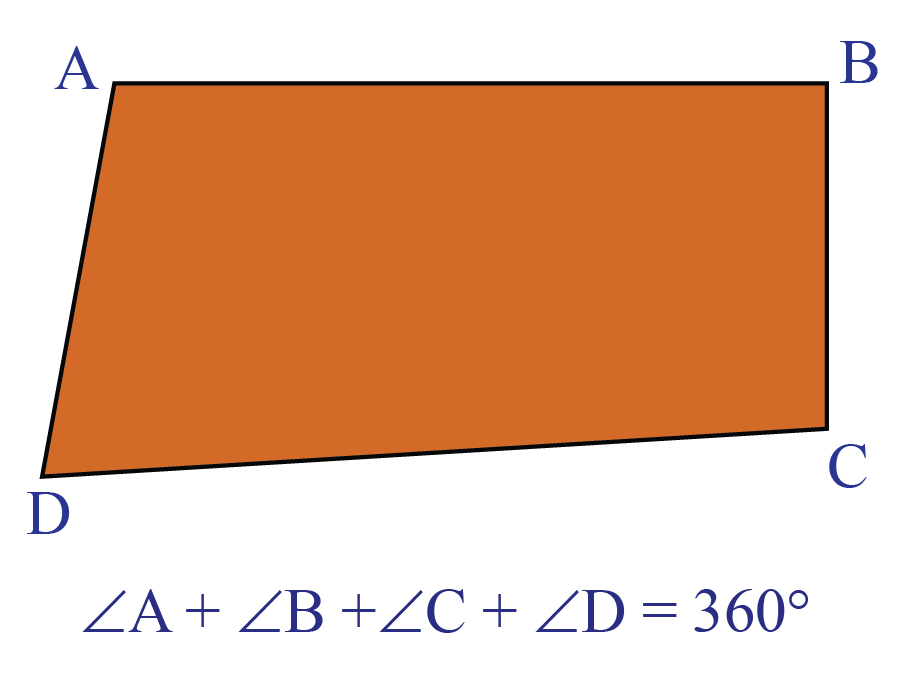But what is the sum of the interior angles of a pentagon, hexagon, heptagon, etc?

Don't you think it would have been easier if there was a formula to find the sum of the interior angles of any polygon?

Well, your wish is granted!

This is the formula to find the sum of the interior angles of a polygon of $$n$$ sides:

 Sum of interior angles = 180(n-2)$$^\circ$$

Using this formula, let us calculate the sum of the interior angles of some polygons.

Polygon

Number of sides, $$\mathbf{ n}$$ Sum of Interior Angles

Pentagon

$$5$$ $$180(5-2) = \mathbf{540^\circ}$$

Hexagon

$$6$$ $$180(6-2) = \mathbf{720^\circ}$$

Heptagon

$$7$$ $$180(7-2) = \mathbf{900^\circ}$$

Octagon

$$8$$ $$180(8-2) = \mathbf{1080^\circ}$$

You can observe this visually using the following illustration.

You can choose a polygon and drag its vertices.

You can then observe that the sum of all the interior angles in a polygon is always constant.

## Finding an Unknown Interior Angle

We can find an unknown interior angle of a polygon using the "Sum of Interior Angles Formula".

For example:

Let us find the missing angle $$x^\circ$$ in the following hexagon.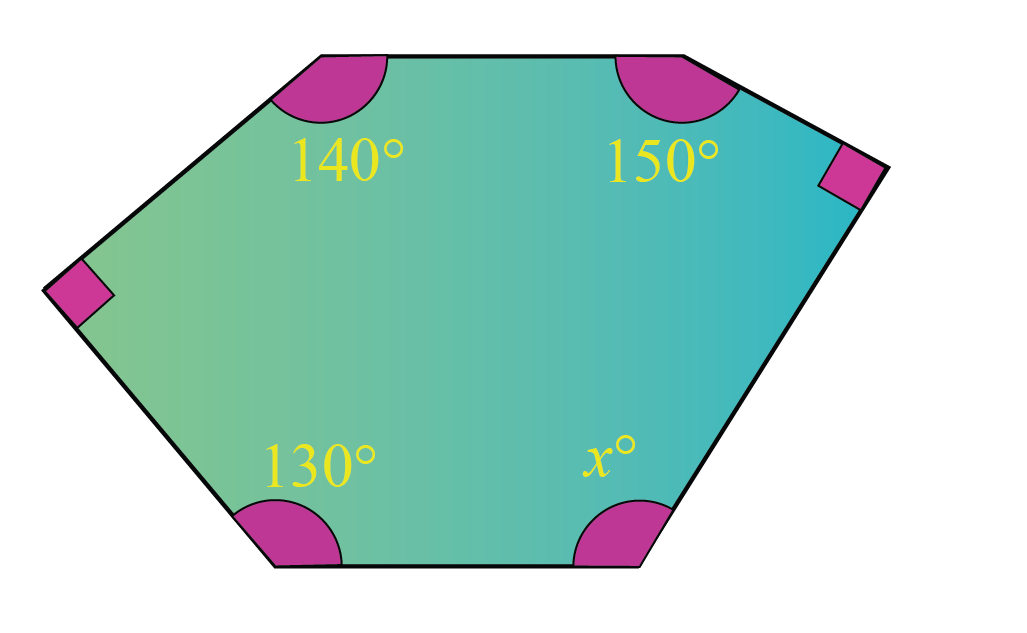From the above table, the sum of the interior angles of a hexagon is 720$$^\circ$$

Two of the interior angles of the above hexagon are right angles.

Thus, we get the equation:

$90 + 90 + 140+150+130+x=720$

Let us solve this to find $$x$$.

\begin{align} 600 + x &= 720\\[0.2cm]x&=120 \end{align}

Thus, the missing interior angle is:

$$x^\circ = 120^\circ$$

## Finding the Interior Angles of Regular Polygons (with Illustration)

A regular polygon is a polygon that has equal sides and equal angles.

Here are some examples of regular polygons: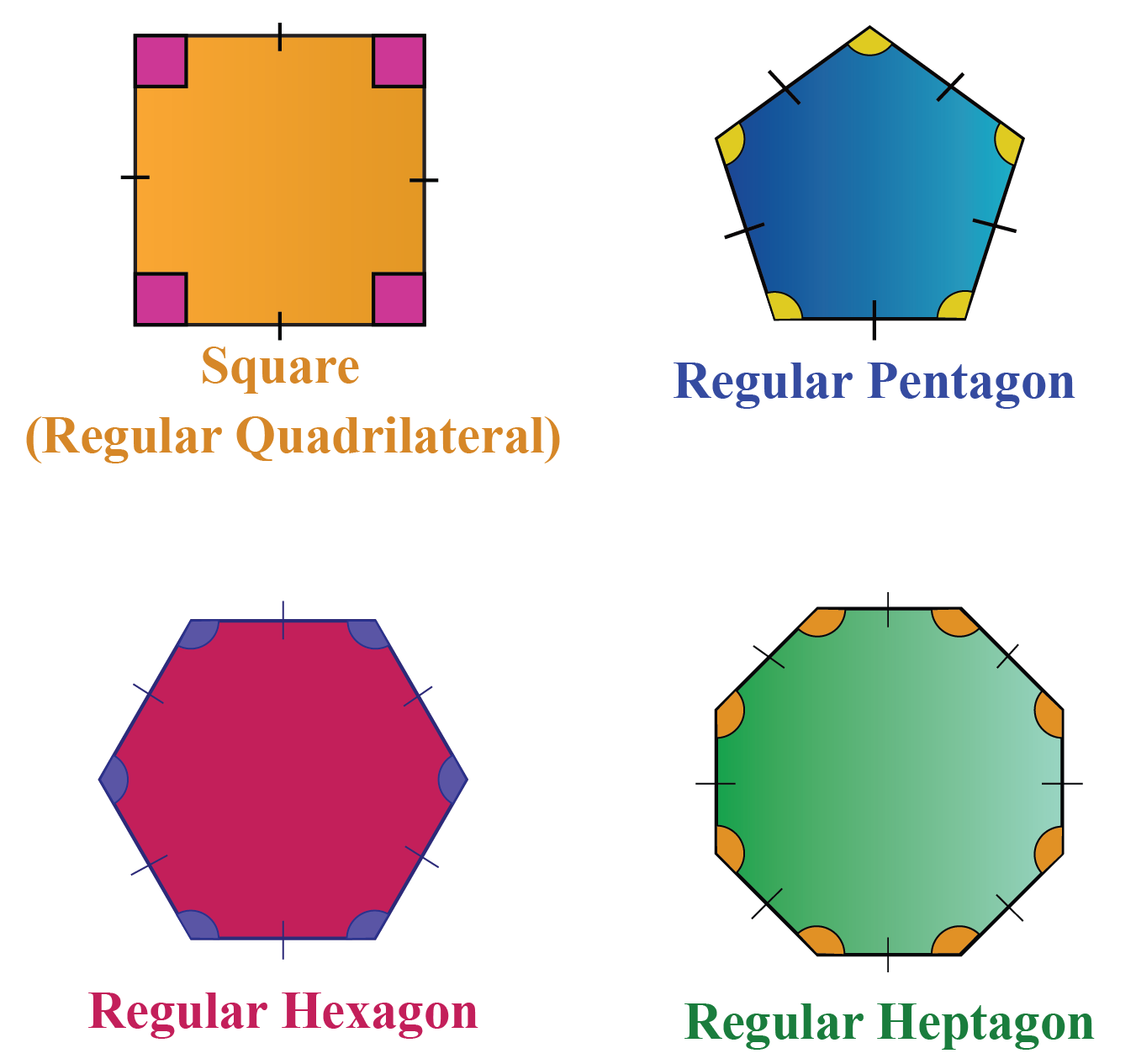We already know that the formula for the sum of the interior angles of a polygon of $$n$$ sides is $$180(n-2)^\circ$$

There are $$n$$ angles in a regular polygon with $$n$$ sides/vertices.

Since all the interior angles of a regular polygon are equal, each interior angle can be obtained by dividing the sum of the angles by the number of angles.

i.e.,

 Each Interior Angle = $$\mathbf{\left(\dfrac{180(n-2)}{n} \right)^\circ}$$

Let us apply this formula to find the interior angle of a regular pentagon.

We know that the number of sides of a pentagon is $$n=5$$

Each interior angle of a regular pentagon can be found using the formula:

$\left(\!\dfrac{ 180(n-2)}{n} \!\right)^\circ \!\!=\!\! \left(\!\dfrac{ 180(5-2)}{5} \!\right)^\circ\!\!=\!\!108^\circ$

Thus, a regular pentagon will look like this: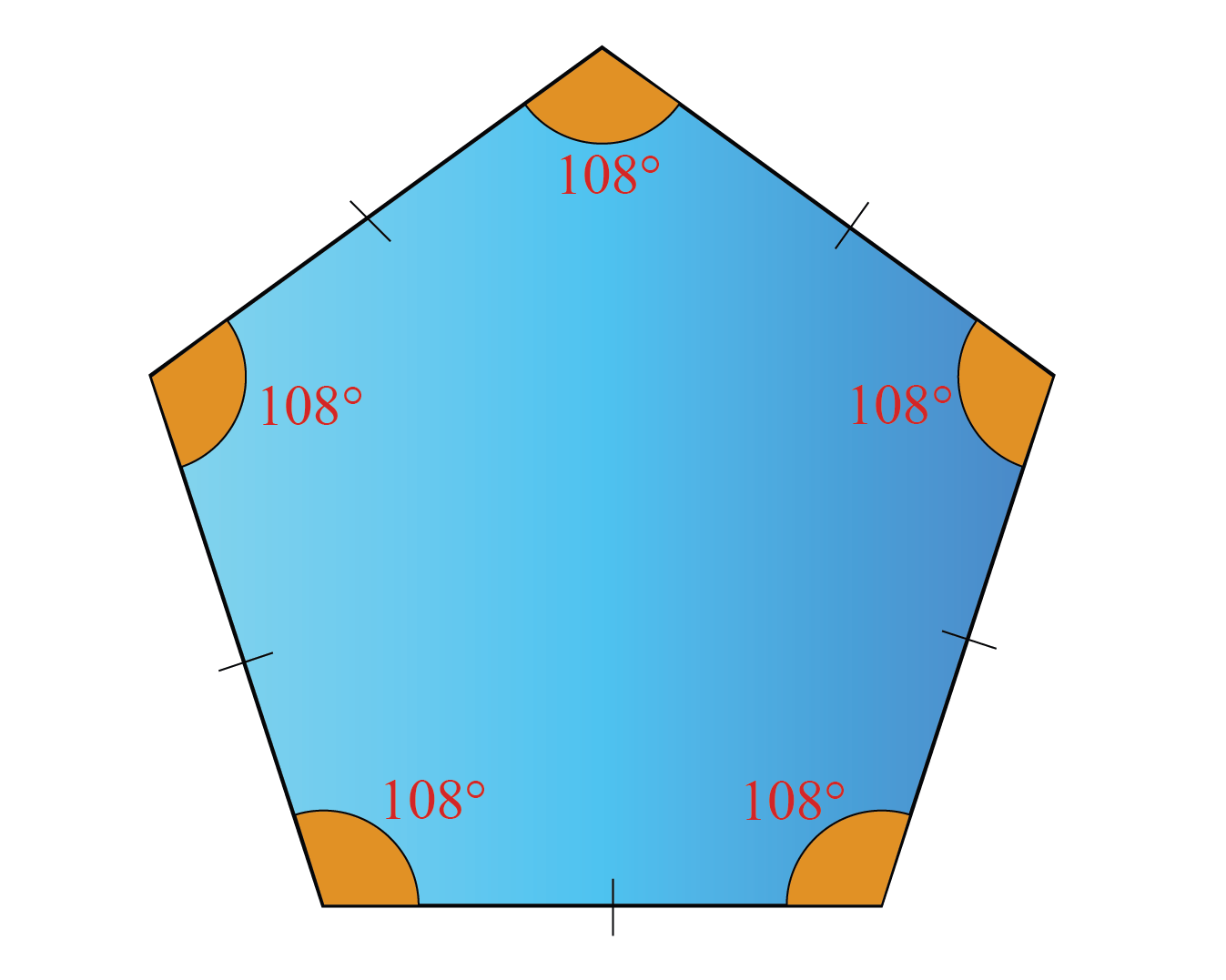Would you like to see the interior angles of different types of regular polygons?

Here is an illustration for you to try.

You can move the slider to select the number of sides in the polygon and then click on "Go".

## Alternate Interior Angle Theorem (with Illustration)

Suppose two parallel lines are intersected by a transversal, as shown below: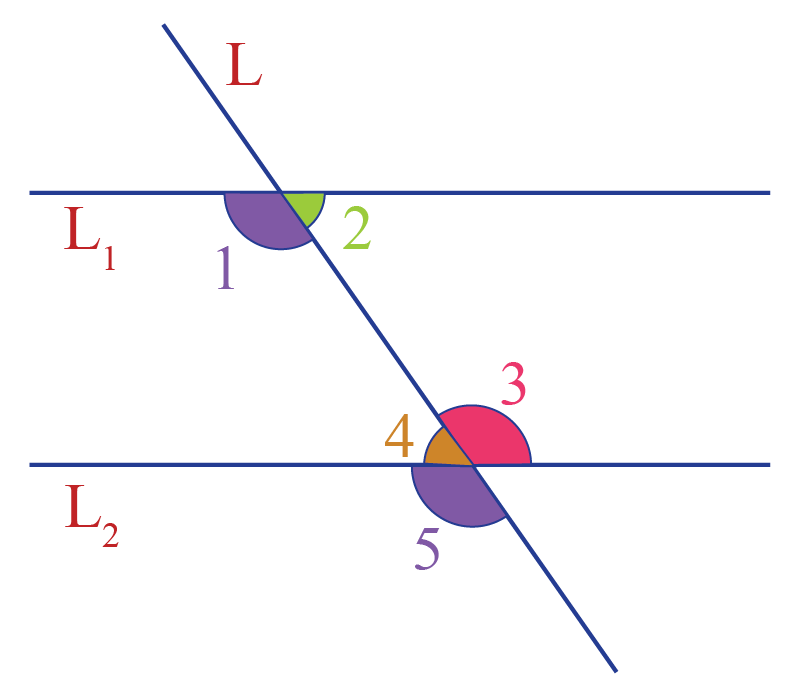What is the relation between any pair of alternate interior angles?

This relation is determined by the "Alternate Interior Angle Theorem"

### Alternate Interior Angle Theorem

When a transversal intersects two parallel lines, each pair of alternate interior angles are equal.

Conversely, if a transversal intersects two lines such that a pair of interior angles are equal, then the two lines are parallel.

### Proof :

Refer to the figure above.

We have:

\begin{align} \angle 1 &= \angle 5 \text{ (corresponding angles)} \\[0.3cm] \angle 3 &= \angle 5 \text{ (vertically opposite angles)} \end{align}

Thus,

$\angle 1 = \angle 3$

Similarly, we can prove that $$\angle 2$$ = $$\angle4$$

Proof of Converse

Conversely, suppose that

\begin{align}\angle 1&= \angle 3 & \rightarrow (1) \end{align}

We have to prove that the lines are parallel.

As $$\angle 3$$ and $$\angle 5$$ are vertically opposite angles,

\begin{align}\angle 3 & = \angle 5 & \rightarrow (2) \end{align}

From (1) and (2),

$\angle 1 = \angle 5$

Thus, a pair of corresponding angles is equal, which can only happen if the two lines are parallel.

Hence, the alternate interior angle theorem is proved.

Would you like to observe visually how the alternate interior angles are equal?

Here is an illustration for you to test the above theorem.

You can change the angles by moving the "Red" dot.

Choose "1st Pair" (or) "2nd Pair" and click on "Go".

## Co-Interior Angle Theorem (with Illustration)

What about any pair of co-interior angles?

The relation between the co-interior angles is determined by the co-interior angle theorem.

### Co-Interior Angle Theorem

If a transversal intersects two parallel lines, each pair of co-interior angles are supplementary (their sum is 180$$^\circ$$)

Conversely, if a transversal intersects two lines such that a pair of co-interior angles are supplementary, then the two lines are parallel.

### Proof :

Refer to the following figure once again:We have:

\begin{align} \angle 1& = \angle 5 \;\;\;\text{ (corresponding angles)} \\[0.3cm]\angle 5 + \angle4& = 180^\circ \;\text{ (linear pair)}\end{align}

From the above two equations, $\angle 1 + \angle4 = 180^\circ$

Similarly, we can show that $\angle 2 + \angle 3 = 180^\circ$

Converse:

Conversely, let us assume that

\begin{align}\angle 1 + \angle4 &= 180^\circ & \rightarrow (1) \end{align}

Since $$\angle 5$$ and $$\angle 4$$ forms linear pair,

\begin{align}\angle 5 + \angle4 &= 180^\circ & \rightarrow (2) \end{align}

From (1) and (2),

$\angle 1 = \angle 5$

Thus, a pair of corresponding angles is equal, which can only happen if the two lines are parallel.

Hence, the co-interior angle theorem is proved.

Would you like to observe visually how the co-interior angles are supplementary?

Here is an illustration for you to try.

You can change the angles by clicking on the purple point and click on "Go".

Geometry
Geometry
grade 9 | Questions Set 2
Geometry